## The microwaves in a microwave oven are produced in a special tube called a magnetron. The electrons orbit the magnetic field at 2.4 GHz, and

Question

The microwaves in a microwave oven are produced in a special tube called a magnetron. The electrons orbit the magnetic field at 2.4 GHz, and as they do so they emit 2.4 GHz electromagnetic waves.

What is the magnetic field strength?

If the maximum diameter of the electron orbit before the electron hits the wall of the tube is 2.5 cm,what is the maximum electron kinetic energy?

in progress 0
6 months 2021-08-02T21:34:59+00:00 1 Answers 24 views 0

The magnetic field strength is 0.086 T

The maximum kinetic energy is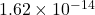J

Explanation:

Given:

Frequency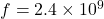Hz

(A)

The magnetic field related to cyclotron is given by,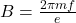Where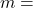mass of electron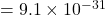kg,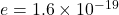C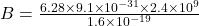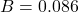T

Therefore, the magnetic field strength is 0.086 T

(B)

Diameter of orbit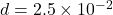m

Radius of orbit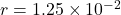m

The maximum kinetic energy is given by,

KE =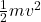Where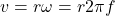KE =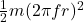KE =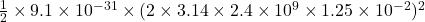KE =J

Therefore, the maximum kinetic energy isJ